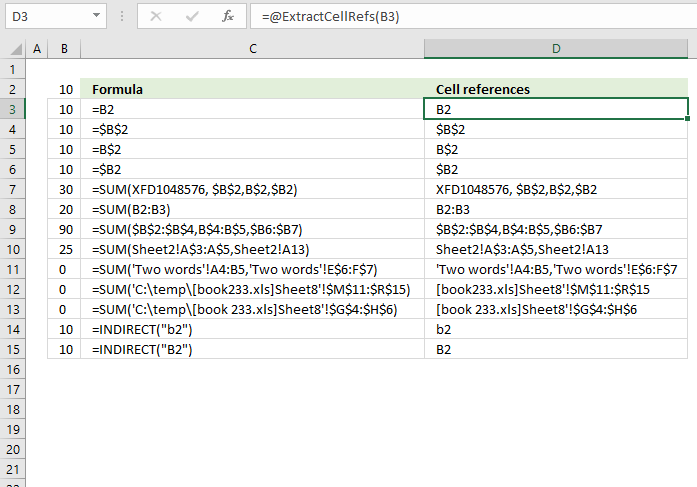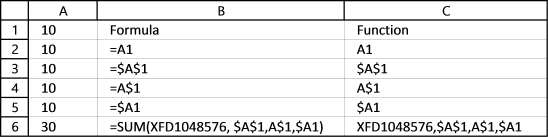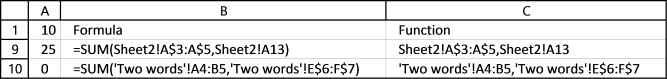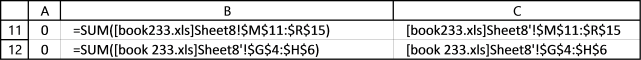Author: Oscar Cronquist Article last updated on June 15, 2021This article demonstrates a User Defined Function that allows you to extract cell references from a formula in a given cell.

This is possible using a regular expression, a regular expression is a sequence of characters that define a search pattern, according to Wikipedia.

## 1. Extract cell references from a formula (User Defined Function)

This is the custom function I am using to extract cell references from a formula.

```'Name User Defined Function (UDF) and specify parameter
Function ExtractCellRefs(c As Range) As String
'Webpage: https://www.get-digital-help.com/extract-cell-references-from-a-formula/
'Save pattern to variable regexpattern, change this before running the UDF
regexpattern = ""

'Evaluate regex pattern against range variable c
With CreateObject("vbscript.regexp")
.Global = True
.MultiLine = True
.IgnoreCase = False
.Pattern = regexpattern

'Save to variable results
Set Results = .Run(c.Formula)
End With

'Check if count is not equal to 0 (zero)
If Results.Count <> 0 Then

'With .. With End statement
With Results

'For ... Next statement
For d = 0 To .Count - 1

'Add item to variable Rstr
Rstr = Rstr & .Item(d) & ","
Next
End With

'Remove last character from string and return string to worksheet
ExtractCellRefs = Left(Rstr, Len(Rstr) - 1)

'Continue here if count is equal to zero
Else

'Return "No Matches" to worksheet
ExtractCellRefs = "No Matches"
End If

End Function

```

## 2. Building the regular expression

The tricky part is the regular expression and I am a beginner at this, feel free to simplify my expression.

A cell reference can be anything from A1 to XFD1048576 so to match that the reg exp becomes:

[a-zA-Z]{1,3}[0-9]{1,7}

#### Step 1 - Brackets match any specified character

You can define a range between A to Z using a hyphen.

[a-zA-Z] matches any letter from A to Z, both upper case and lower case.

#### Step 2 - Curly braces define the number of characters

[a-zA-Z]{1,3} matches 1 or up to 3 lower and upper case letters from A to Z. Example, XFD1048576 contains three letters.

#### Step 3 - Match a specified number of digits

[0-9]{1,7} matches 1 or more up to 7 digits from 0 to 9. Example, XFD1048576 contains 7 digits.

## 3. Absolute and relative cell references - regexA cell reference can also be absolute or relative or both and the \$ sign tells which it is.

\\$?[a-zA-Z]{1,3}\\$?[0-9]{1,7}

#### Step 1 - Dollar sign is a special character

The dollar sign is a special character in a regular expression, we need to use the backslash to make an expression that matches the dollar sign itself.

\ (backslash) escapes the character that follows

\\$ allows us to use the character \$, if I had not used the \ (backslash) \$ (dollar sign) had been taken for a match at end of the string, I don't want that to happen.

#### Step 2 - Combine dollar sign with the regex pattern so far

\\$? the question mark matches zero or one of the patterns defined before it, in this case, \$ (dollar sign)

The picture below shows the matches for the above expression \\$?[a-zA-Z]{1,3}\\$?[0-9]{1,7}It also works for this simple formula: =SUM(XFD1048576, \$A\$1,A\$1,\$A1), it returns these cell references: XFD1048576,\$A\$1,A\$1,\$A1

## 4. Cell reference to a cell rangeThe above regular expression finds only cell refs to a single cell, a cell reference can also point to a cell range. How do we find a solution to that?

A cell ref to a single cell looks like this =A1, a cell ref to a cell range may look like this =A1:C3

\\$?[a-zA-Z]{1,3}\\$?[1-9]{1,7}(:\\$?[a-zA-Z]{1,3}\\$?[1-9]{1,7})?

The new part is

(:\\$?[a-zA-Z]{1,3}\\$?[0-9]{1,7})?

#### Step 1 - Parentheses creates a group

( (parentheses)  groups an expression

#### Step 2 - A colon is used if the cell reference points to a cell range

: a cell ref to a cell range contains a colon :

#### Step 3 - Combine parts

\\$?[a-zA-Z]{1,3}\\$?[0-9]{1,7} is the same as before, it matches letters and digits

(:\\$?[a-zA-Z]{1,3}\\$?[0-9]{1,7})  the parentheses groups the expression

#### Step 4 - Question mark matches the group

(:\\$?[a-zA-Z]{1,3}\\$?[0-9]{1,7})? the question mark matches zero or one of the pattern defined before it, in this case, the group.## 5. Cell references to other sheets

A cell reference to another sheet always ends with a ! (exclamation mark), the question mark matches zero or one of the patterns defined before it, in this case, the ! (exclamation mark)

This is a new group so I am leaving out the previous expression for now, I will add it later. The regular expression is now !?

The sheet name may have lower and upper letters from A to Z and also numbers 0 to 9, the regular expression is [a-zA-Z0-9]{1,99}!?

If there is a blank space in the sheet name Excel automatically surrounds the sheet name with two ' (apostrophe character), the expression becomes '?[a-zA-Z0-9]{1,99}'?!?

\s is any space character, this is what we have now '?[a-zA-Z0-9\s]{1,99}'?!?

'? the question mark matches zero or one of the patterns defined before it, in this case, the ' (apostrophe character)## 6. Cell references to other workbooks

There may also be cell references to other workbooks, it would be nice to find them as well.

A reference to a cell range in another workbook has the workbook name surrounded by these characters [].

('?[a-zA-Z0-9\s\[\]]{1,99})?'?!? , \ (backslash) escapes the character that follows in this case \[\]

The file name has a dot between the file name and the extension, ('?[a-zA-Z0-9\s\[\]\.]{1,99})?'?!?The final expression is

('?[a-zA-Z0-9\s\[\]\.]{1,99})?'?!?\\$?[a-zA-Z]{1,3}\\$?[0-9]{1,7}(:\\$?[a-zA-Z]{1,3}\\$?[0-9]{1,7})?

Can it be made smaller?

### Final thoughts

I am sure there are characters allowed in a filename or sheet name that I have not considered in this post but I believe it will be easy to add those as well.

This does not take care of named ranges in a formula but it would not be hard to build a list of named ranges and then check if the formula contains named ranges.

Don't forget to use the regular expression in the UDF. Replace this line:

```regexpattern = ""
```

with this:

```regexpattern = "'?([a-zA-Z0-9\s\[\]\.])*'?!?\\$?[a-zA-Z]+\\$?[0-9]+(:\\$?[a-zA-Z]+\\$?[0-9]+)?"
```

### Get the Excel fileExtract-cell-refs-in-formulav2.xlsm